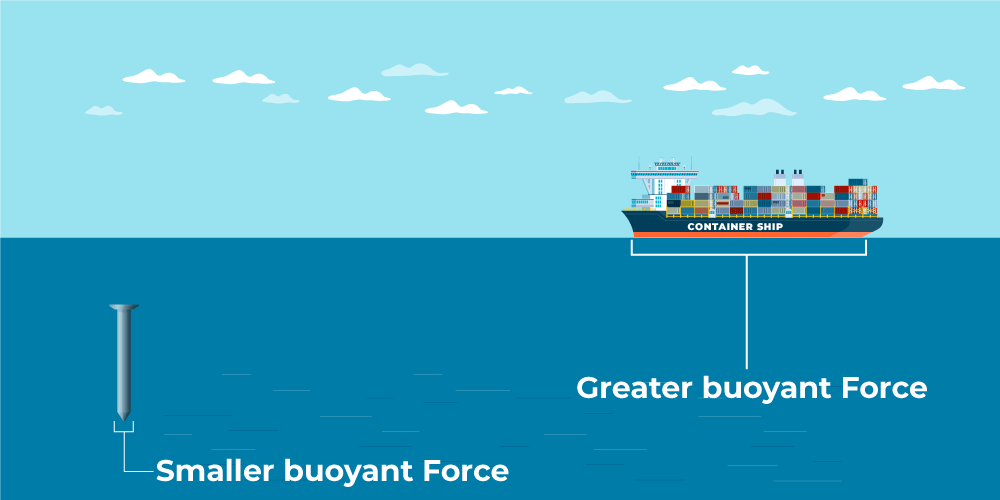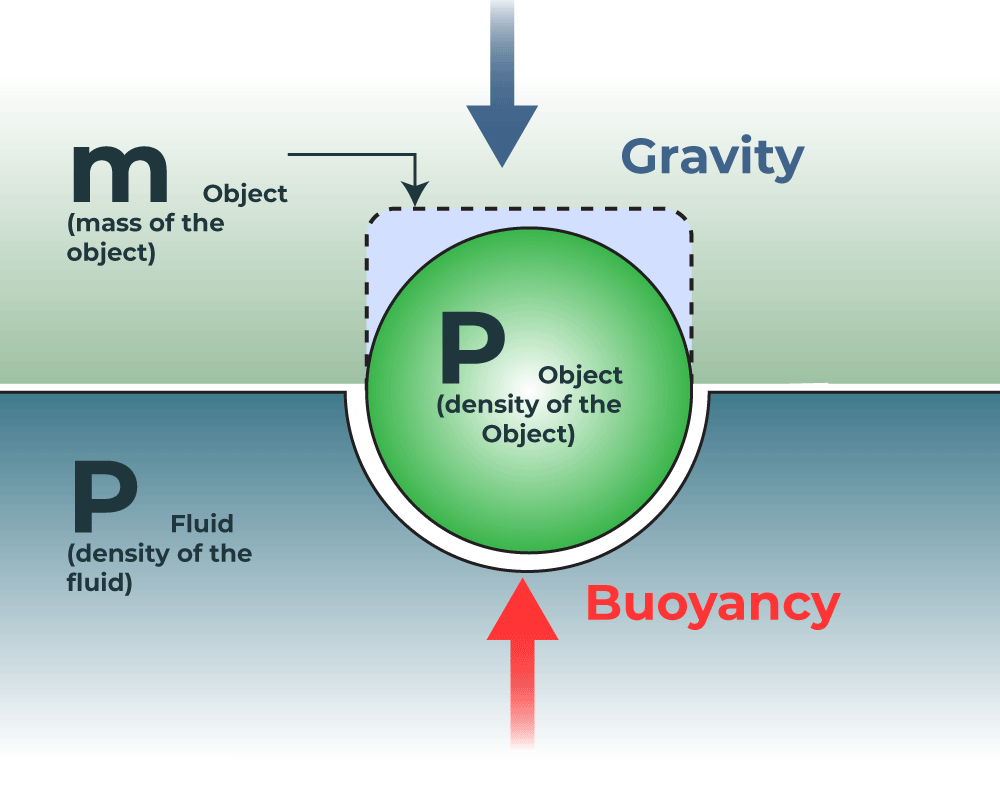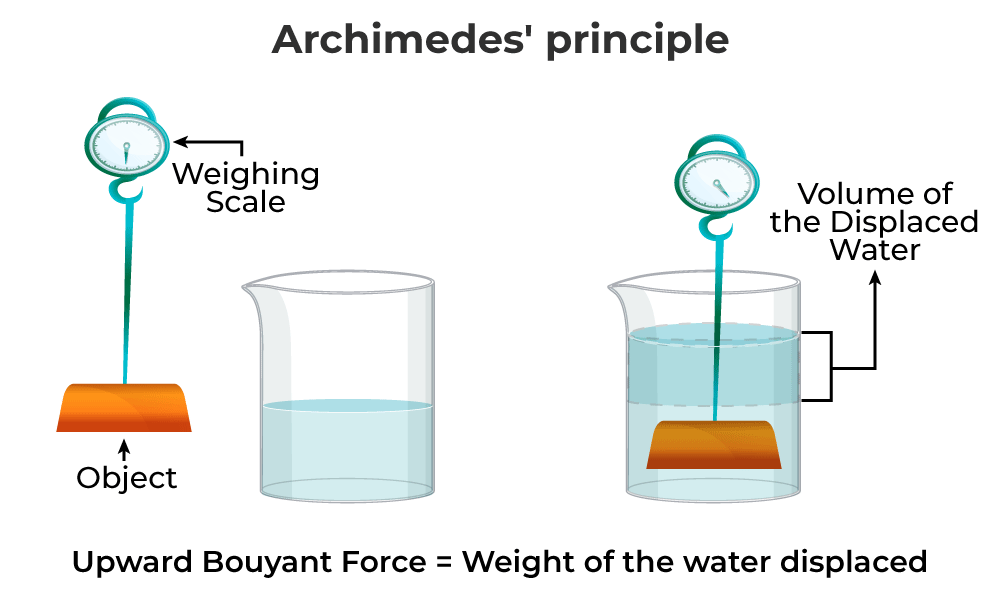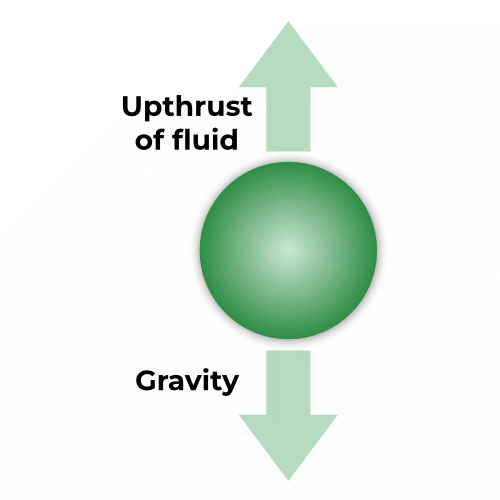GFG App
Open AppBrowser
Continue

Buoyancy is a phenomenon due to buoyant force that causes an object to float. When you put an object in a liquid, an upward force is exerted on the object by the liquid. This force is equal to the weight of the liquid that has been displaced. The amount of liquid that has been displaced depends upon the density and the volume of the object immersed in the liquid. Have you ever wondered why an iron nail sinks in water, but a ship made up of iron floats? And why does an iron ball sink but a plastic ball of the same size floats in water? These wonders happen due to the phenomenon known as Buoyancy. Let’s learn the buoyancy definition, buoyancy equation, and its examples in detail,

## What is Buoyant Force?

Buoyant force can be defined as an upward force exerted on an object that is completely or partially submerged in liquid. The unit of the buoyant force is Newton. Buoyancy force depends upon two factors:

1. Amount (Volume) of liquid displaced by the object
2. The density of the object.

In the first example, the iron nail has less volume and displaces a very less amount of water, so there is less buoyant force (upward force), and therefore sinks. Whereas ships have more volume, displace more water, and therefore have greater buoyant force (upward force) by water, and it floats. When talking in terms of relative density, if the relative density is less than 1, the object floats in water, and if the relative density is more than 1, the object sinks.In the second example, the iron ball has more density and, therefore, greater gravitational force (downward force) than the buoyant force applied by the liquid. Hence it sinks. Whereas plastic ball is hollow and very dense, so smaller gravitational force (downward force) than the buoyant force (upward force) applied by liquid, and it floats. Boats, submarines, lifeboats, life jackets, and swimming work on the principle of buoyancy.

## What causes Buoyant Force?

An object when immersed in a liquid experiences buoyant force. This force is upward in the direction opposite to the gravitational force, this is responsible for the reduced weight of the object inside the fluid. It’s known that the pressure increases with the depth of the fluid. The pressure at the bottom of the object is greater than the pressure experienced at the top, this difference creates the net force experienced by the object inside the liquid, which is called buoyant force.## Archimedes’ Principle

The physical law of buoyancy was given by the Greek mathematician and inventor Archimedes. Archimedes’ principle states that the upward buoyant force that is exerted on a body immersed in a fluid, whether fully or partially, is equal to the weight of the fluid that the body displaces. In the image given below, fluid pressure opposes gravity, and actual gravitational forces decrease therefore given by:

Apparent weight = Weight of object (in the air) – Thrust force (buoyancy)### Formula for Archimedes’ Principle

Archimedes’ law is mathematically written as:

Fb = ρ × g × V

Where,

• Fb is the buoyant force.
• ρ is the density the fluid.
• V is the submerged volume.
• g is the acceleration due to gravity.

### Derivation of the Formula

After rearranging the formula ρV is the density of the displaced fluid multiplied by the volume of the displaced fluid, and we know the mass, density, and volume relation.

m = ρV

That means the term ρV corresponds to the mass of the displaced fluid.

Fb = ρ × g × V

Fb = mg i.e., The mass of the displaced fluid times the magnitude of the acceleration due to gravity is just the weight of the displaced fluid.

Fb = W

The above equation, when stated in words called Archimedes’ principle. Assuming Archimedes’ principle to be reformulated

Apparent immersed weight = weight – Weight of displaced fluid

Then inserted into the quotient of weights, which has been explained by the mutual volume.The density of the immersed object relative to the density of the fluid can be calculated:Fb = PA = g ρ V = ρ g h A ⇢ (i)

• P = pressure
• Fb = force of buoyancy in Newton,
• A = Area in meter square,
• g = acceleration due to gravity,
• h = Height at which force acts taken from the surface
• p = density of the fluid,
• V = volume of the object inserted into the fluid.

Fb = Wa – Wf ⇢ (ii)

• Fb = force of buoyancy
• Wa = Weight of the object in the air
• Wf  = Weight of the object when it is immersed in the fluid

Using (l) and (ll),

g ρ V = Wa – Wf ⇢ (iii)

If the object is not sinking, then Fg – Fb:

Mg = ρ g V

### Demonstration of Buoyant Force

An object that is partly or completely submerged experiences a higher pressure on the bottom surface than on its top surface. This force is called upthrust. When we put something on the water body’s surface, it displaces some of the fluid. The upthrust force is equal to the weight of the fluid displaced by the object.A floating object is stable if it tends to restore itself to an equilibrium position after a small displacement. For example, floating objects will generally have vertical stability. As if the object is pushed down slightly, this will create a greater buoyancy force, which, unbalanced by the weight force, will push the object back up. Given a small angular displacement, the vessel may return to its original position (stable), move away from its original position (unstable), Or remain where it is (neutral). Rotational stability depends on the relative lines of action of forces on an object. The upward buoyancy force on an object acts through the center of buoyancy (CB), being the centroid of the displaced volume of fluid. The weight force on the object acts through its center of gravity (CG).

The buoyant object will be stable if the center of gravity is beneath the center of buoyancy because any angular displacement will then produce a ‘righting moment’. The stability of a buoyant object at the surface is more complex, and it may remain stable even if the center of gravity is above the center of buoyancy, provided that when disturbed from the equilibrium position, the center of buoyancy moves further to the same side that the center of gravity moves, thus providing a positive righting moment.

### What is Upthrust?

Any object placed in a fluid receives an upward force called Upthrust. The object is pushed in an upward direction, therefore, named Upthrust. An upthrust is a force that is applied by the water, and one other force is also acting on the body due to the mass of the body which is known as gravitational force. Gravitational force is acting in the opposite direction of upthrust force, Upthrust force acts in the vertically upward direction, and the Gravitational force acts in the vertically downward direction. The unit of upthrust is Newton

## Why does an Object float or sink in the water?

Any liquid can be considered a concoction of many overlying layers. These layers are lying one over another and have fluctuating pressure. Since the pressure increases with depth, thus the pressure at the bottom will be more than the pressure at the top which will cause the buoyant force. The difference in pressure at these layers gives rise to the buoyant force. The magnitude of the force is given by the weight of the liquid displaced by the object submerged in the liquid. Due to this phenomenon, objects float in the water.

## Types of Buoyancy

There are three types of buoyancy:

• Positive buoyancy: When the weight of an object is lighter than the fluid it displaces is called positive buoyancy. For example, a boat that weighs (3000 kg) but displaces (4500 kg) of water will easily float
• Negative buoyancy: When the weight of an object is greater than the fluid it displaces is called positive buoyancy. For example, an iron nail may weigh 27 grams, but if it only displaces 17 grams of water, it will sink.
• Natural buoyancy: When the weight of an object is equal to the fluid it displaces. For example, a submarine can adjust its weight by adding or expelling water in special tanks called ballast tanks is an example of natural buoyancy.

## Application of Buoyancy

The buoyancy force is a phenomenon that can be observed in a lot of places in real life and in nature. The force makes it possible for us to swim, and build machines like ships and planes. Let’s learn about some examples in more detail,

### Hot Air Balloon

The atmosphere is filled with air, which is also a certain type of fluid. Hot air balloons leverage the buoyancy effect. Air exerts a force on the balloon. The balloon rises up when the force exerted is less than the weight of the balloon and conversely, it descends when the force exerted is less than the weight of the balloon. Sometimes hot air balloons become stationary in mid-air, in that particular case, the weight of the balloon becomes equal to the force applied by the displaced liquid (air).

### Ship

• The boat is designed in a way so that the shape of the boat is hollow. Due to the hollow shape, the density becomes less than the density of the sea. The volume of water displaced by the boat is equal to the weight of the boat and this helps the ship to float.
• Fishes stay buoyant underwater. Fishes have a special organ called a swim bladder that is usually filled with gases thus making the body lighter. This enables the fish to go up.
• Floatation of submarines in the water.
• To find the volume of the body.
• To keep swimmers on top of the water body.

## Solved Examples on Buoyancy

Example 1: Find the volume of the immersed object if M is 10000 kg.

Solution:

ρ = 997 kg/m3

10000 kg × g = g × 997 kg/m3 V

1000 kg = 997 kg/ m3 VExample 2: A block of wood with length = 3.2 m, width = 0.8 m, and height = 0.6 m. The density of water is 1000 kg/m3. If the block is placed in the water, what is the buoyant force? Acceleration due to gravity is 10 N/kg.

Solution:

Volume of the block (V) = length × width × height = 3.2 × 0.8 × 0.6 = 1.53 m3

Density = 1000 kg/m3

Gravity = 10 N/Kg

F = density × gravity × volume

F = 1000 × 10 × 1.53 = 15300N

F = 15300N

Example 3: The weight of an object in the air is 108 N. The object is placed in a liquid. The increase in the volume of liquid is 1.8 m3. If the specific weight of the liquid is 10 N/m3, what is the weight of the object in the liquid?

Solution:

Object’s weight in liquid = object’s weight in air – buoyant force

Object’s weight in liquid = 108 N – buoyant force

F = ρ g V

The density of liquid is 1 kg/m3

F = ρgV = 1 × 10 × 1.8 = 18 kg m/s2 = 18N.

Object’s weight in fluid = 100 N – 18 N = 82N

Example 4: An object weighs 12N in air. When immersed fully in water, it weighs only 9N. What would be the weight of the liquid displaced by the object?

Solution:

According to Archimedes’s law:

Apparent weight = Weight of object (in the air) – Thrust force (buoyant force)

Apparent weight = 9N

Weight of object (in the air) = 12N

Thrust force (buoyant force) = ?

Apparent weight = 12N – Fb

Fb = 12N – 9N = 3N

Example 5: A block of wood with length = 3.2 m, width = 0.8 m, and height = 0.6 m. The density of water is 1000 kg/m3. If the block is placed in the water, what is the buoyant force? Acceleration due to gravity is 10 N/kg.

Solution:

F = ρ g V

F = 1000 × 10 × 1.58 = 15840N

Example 6: The weight of an object in the air is 108 N. The object is placed in a liquid. The increase in the volume of liquid is 1.8 m3. If the specific weight of the liquid is 10 N/m3, what is the weight of the object in liquid?

Solution:

Object’s weight in liquid = object’s weight in air – buoyant force

Object’s weight in liquid = 108 N – buoyant force

FA = ρ g V

The density of liquid is 1 kg/m3

FA = ρgV = (1kg/m3)(10m/s2)(1.8m3) = 18kgm/s3 = 18N

Object’s weight in fluid = 100 N – 18 N = 82N

Example 7: A piece of marble tile weighs 285 g in air. If its density is 3.5 g/cc, what will be its weight in water?

Solution:

Weight in air = 285 g

Volume = 285g /(3.5 g/cc) = 81.4 cc

Weight in water = 285 g – 81.4g = 203.6 g

## FAQs on Buoyancy

Question 1: What causes buoyancy?

When an object is immersed in water, pressure is exerted on the object, known as buoyancy. The force acting upwards is called buoyant force.

Question 2: What are the three types of buoyancy?

There are three types of buoyancy:

• Positive buoyancy: When the weight of an object is lighter than the fluid it displaces is called positive buoyancy.
• Negative buoyancy: When the weight of an object is greater than the fluid it displaces is called positive buoyancy.
• Natural buoyancy: When the weight of an object is equal to the fluid it displaces.

Question 3: Why does buoyant force play an important role in swimming?

When a swimmer is inside the water body, it is important that he/she stays on near the surface for safety, this is possible due to buoyant force which is a little more than the pressure exerted from above.

Question 4: What is the difference between buoyancy and buoyant force?

The difference between buoyancy and buoyant force is that buoyant force is the net force exerted upward on an object partially or completely submerged in water. Whereas, buoyancy is the phenomenon caused by the buoyant force. It is the tendency of an object submerged in water.

Question 5: What is the buoyant force equation?

The equation for the buoyant force is defined by Archimedes’ principle. It is given as:

Fb = -ρgV

Where,

• Fb is the buoyant force.
• ρ is the density of the fluid.
• V is the submerged volume.
• g is the acceleration due to gravity.

Question 6: How are density and buoyancy related?